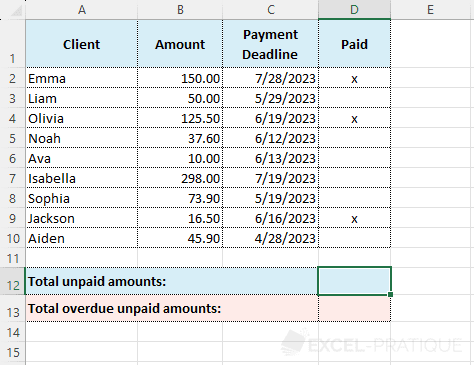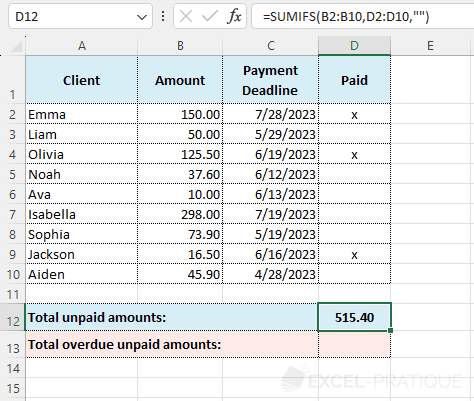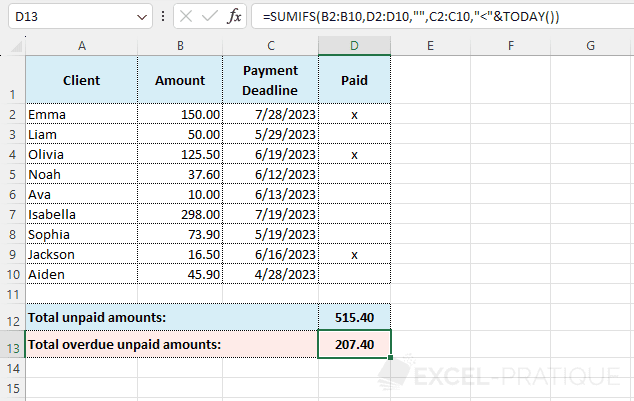# Excel Function: SUMIFS

The SUMIFS function allows you to perform the sum of a range of cells according to several criteria (the SUMIF function on the other hand is limited to a single criteria).

Usage:

`=SUMIFS(Sum_range, Criteria_range1, Criteria1)`

or

`=SUMIFS(Sum_range, Criteria_range1, Criteria1, Criteria_range2, Criteria2, ...)`

## Usage Example

The goal here is to first calculate the sum of unpaid amounts (= 1 criteria) then the sum of overdue unpaid amounts (= 2 criteria):Enter the SUMIFS function:

• Sum_range: the range of cells to add up (here, the amounts)
• Criteria_range1: the range evaluated with Criteria1 (here, the "Paid" column)
• Criteria1: the evaluation criteria (here, `""` to check if the cell is empty)

So, the formula is:

``=SUMIFS(B2:B10,D2:D10,"")``Since there is only one criteria here, the SUMIF function could also have been used (however, be careful with the order of the arguments which is not the same with this function).

To get the sum of overdue unpaid amounts, you have to add a second criteria to the formula that will check if the payment deadline has been exceeded (so, a date less than TODAY):

``=SUMIFS(B2:B10,D2:D10,"",C2:C10,"<"&TODAY())``